[Next]: Optimal control of surface heat treatments
 [Up]: Project descriptions
 [Previous]: Physical modeling and numerical simulation of
[Contents]   [Index]

## Stationary solutions of two-dimensional heterogeneous energy models with multiple species near equilibrium

Collaborator: A. Glitzky , R. Hünlich

Cooperation with: L. Recke (Humboldt-Universität zu Berlin)

Supported by: DFG: Zur Analysis von thermodynamischen Modellen des Stoff-, Ladungs- und Energietransports in heterogenen Halbleitern'' (Analysis of thermodynamic models for the transport of mass, charge and energy in heterogeneous semiconductors)

Description: We consider a stationary energy model for reaction-diffusion processes of electrically charged species with non-local electrostatic interaction. Such problems arise in electrochemistry as well as in semiconductor device and technology modeling (see, e.g., ). But, in contrast to  we now additionally take thermal effects into account. We consider a finite number of species Xi,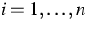(e.g., electrons, holes, dopants, interstitials, vacancies, dopant-defect pairs). Let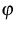and T be the electrostatic potential and the lattice temperature. We denote by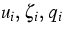the particle density of the i-th species, its electrochemical potential and its charge number. The state equations are assumed to be given by the ansatz (see )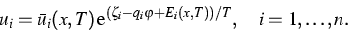We consider a finite number of reversible reactions of the form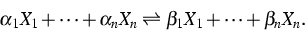The set of stoichiometric coefficients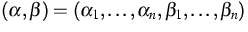belonging to all reactions is denoted by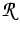.According to the mass-action law the reaction rates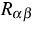are prescribed by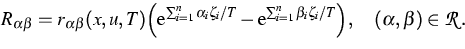Here u means the vector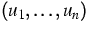.For the particle flux densities ji and the total energy flux density je we make the ansatz (see )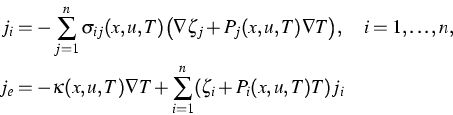with conductivities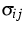,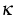fulfilling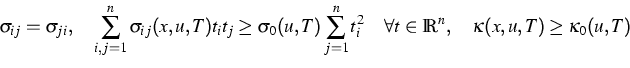where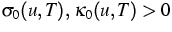for all non-degenerated states u, T. For the fluxes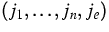and the generalized forces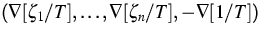, the Onsager relations are fulfilled. The basic equations of the energy model contain n continuity equations for the considered species, the conservation law of the total energy and the Poisson equation,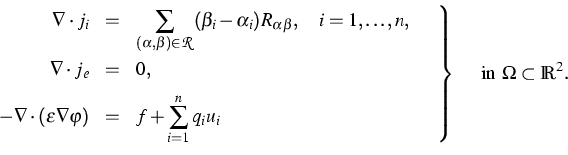(1)
Here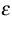is the dielectric permittivity and f represents some fixed charge density. System (1) must be completed by suitable mixed boundary conditions. We introduce the new variables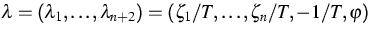.With some functions Hi we can reformulate the state equations in the form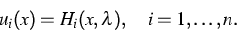Also the reaction ratesare expressed in the new variables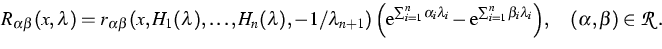Then the stationary energy model can be written as a strongly coupled nonlinear elliptic system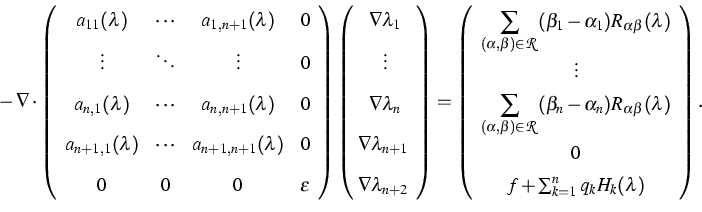(2)
Here we have omitted the additional argument x of the coefficient functions. We assume that the Dirichlet parts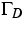and the Neumann parts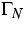of the boundary conditions coincide for all quantities. Then we can formulate the boundary conditions in terms of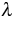,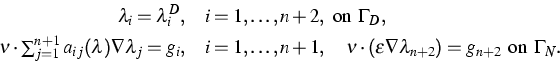(3)
We assume that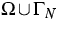is regular in the sense of  and that the boundary values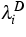,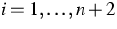, are traces of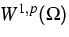functions, p>2. We are looking for solutions of (2), (3) in the form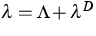.Under weak assumptions on the coefficient functions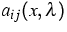and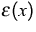(such that heterostructures are allowed) we found W1,q formulations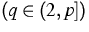for that system of equations,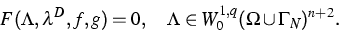If the boundary values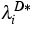,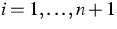,are constants,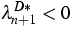and,, correspond to a simultaneous equilibrium of all reactions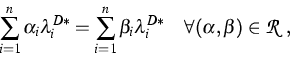and if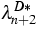,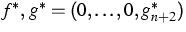are arbitrarily given, then there exists a unique solution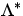of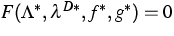.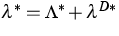is a thermodynamic equilibrium of (2), (3). The operator F is continuously differentiable and the linearization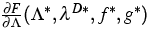turns out to be an injective Fredholm operator of index zero. This follows from results in  and from a regularity result of Gröger in  for systems of elliptic equations with mixed boundary conditions. Therefore we can apply the Implicit Function Theorem and obtain that for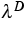near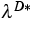,f near f* and g near g* the equation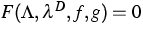has a unique solution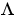near. Thus, near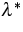there is a locally unique Hölder continuous solutionof (2), (3). For details and the precise assumptions of our investigations see .

Our local existence and uniqueness result for the stationary energy model (2), (3) works in two space dimensions. But let us note that in our model equations cross-terms with respect to all species and temperature are involved.

References:

1. G. ALBINUS, H. GAJEWSKI, R. HÜNLICH, Thermodynamic design of energy models of semiconductor devices, Nonlinearity, 15 (2002), pp. 367-383.
2. A. GLITZKY, Elektro-Reaktions-Diffusionssysteme mit nichtglatten Daten, habilitation thesis, Humboldt-Universität zu Berlin, 2001, Logos Verlag, 2002.
3. A. GLITZKY, R. HÜNLICH, Stationary solutions of two-dimensional heterogeneous energy models with multiple species near equilibrium, in preparation.
4. K. GRÖGER, A W1,p-estimate for solutions to mixed boundary value problems for second order elliptic differential equations, Math. Ann., 283 (1989), pp. 679-687.
5. L. RECKE, Applications of the implicit function theorem to quasi-linear elliptic boundary value problems with non-smooth data, Comm. Partial Differential Equations, 20 (1995), pp. 1457-1479.

 [Next]: Optimal control of surface heat treatments
 [Up]: Project descriptions
 [Previous]: Physical modeling and numerical simulation of
[Contents]   [Index]

LaTeX typesetting by I. Bremer
5/16/2003